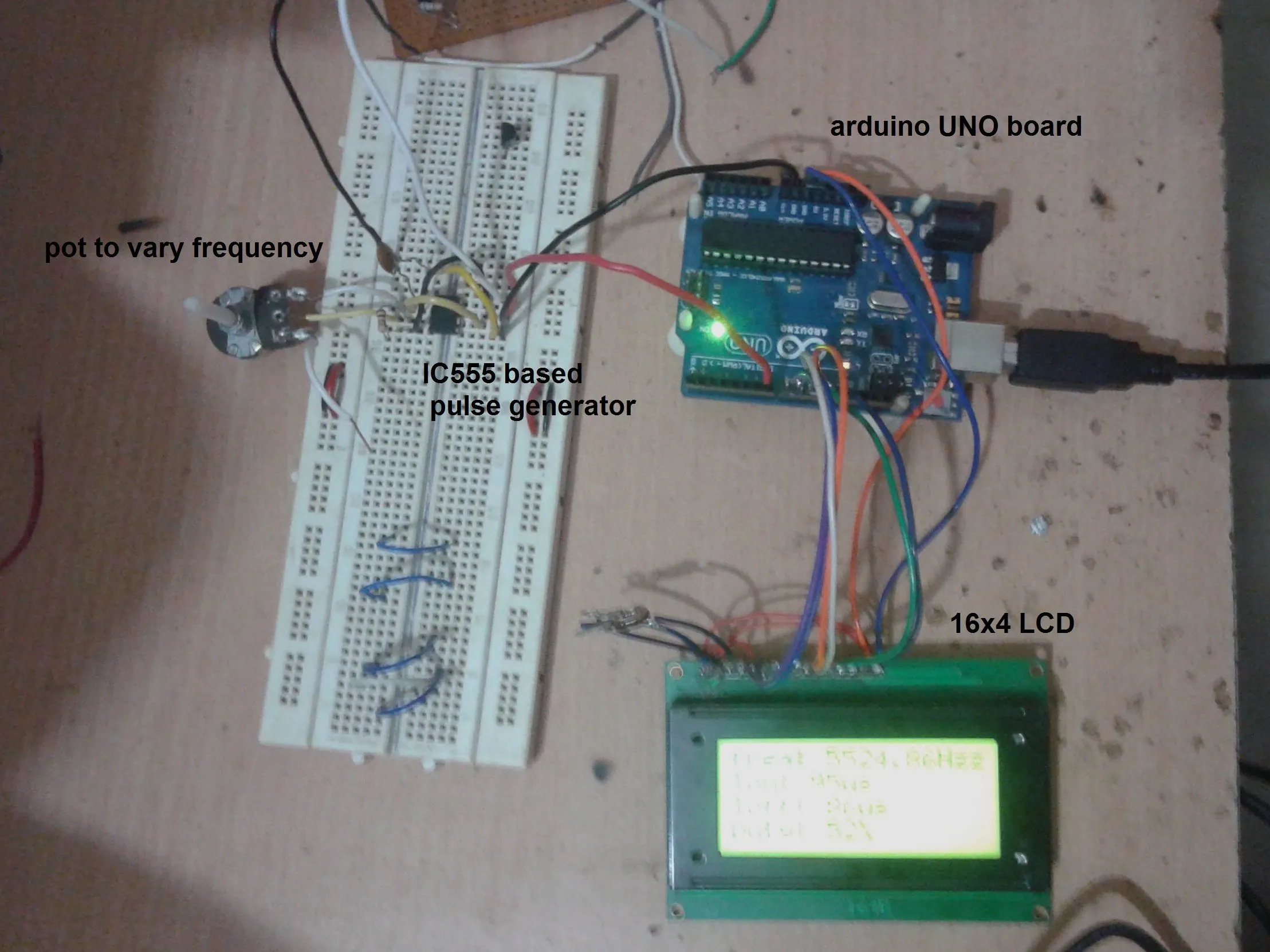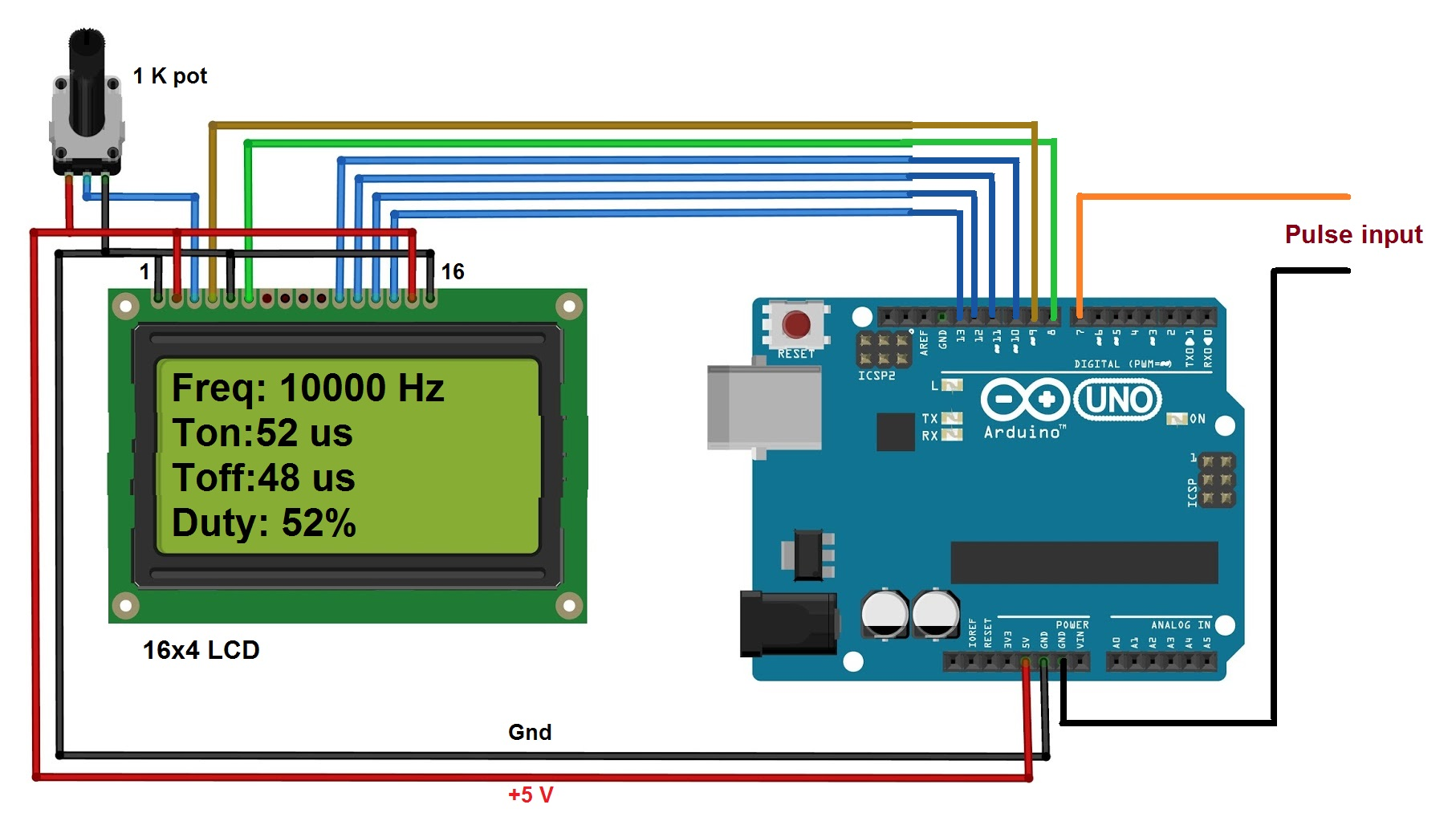Project tutorial# Frequency and Duty Cycle Measurement Using Arduino © GPL3+

Arduino is used to measure frequency and duty cycle of pulses and display them on LCD.

• 38,098 views
• 2 comments
• 17 respects

## About this project

Arduino has several applications. We may find its application in many different fields and areas. It can be used in measurement field also to measure electrical quantities (like voltage, current, power etc) or physical quantities (like temperature, moisture, light intensity, humidity etc) or electronic component values etc.

The given article demonstrates how to measure frequency and duty cycle of pulses using arduino. Frequency measurement is required in so many different applications. In communication field frequency measurement is at most essential. Duty cycle is also an important parameter to measure because it gives % of pulse width – means ON time of pulse. In DC motor speed control and servo motor angle control it is required to measure width of pulse. Also the pulse width is measured to check the symmetry of pulse in some of the application like digital signal receiver, repeaters etc. So let us see how we can use arduino to measure frequency and duty cycle of pulses. In given project, the arduino measures frequency, ON time, OFF time and duty cycle of pulses and displays them on 16x4 LCD

Description:

As shown in above figure there are only two major components in the circuit (1) arduino UNO development board and (2) 16x4 LCD display

· The pulses are directly given as an input to digital pin 7 of arduino

· The Rs and En pins of LCD are connected to digital pins 9 and 8 respectively of arduino board. Rw pin is connected to ground

· Last four data pins D4 – D7 are connected to arduino pins 10, 11, 12 and 13

· The anode pin of back light LED (pin 15) and Vcc pin (2) of LCD are given 5 V supply through arduino board

· Cathode of back light LED (pin 16) and Vss pin (1) are connected to ground

· One1 K pot is connected to Vee pin to vary LCD contras

Circuit operation:

· When arduino board is given supply through USB, four parameters are displayed on LCDas “freq:Ton:Toff:Duty: “on each row as shown

· Now when pulses are fed to pin 7, the arduino first wait for pulse to be high. When it becomes high it calculates the time period (in micro second) for which the pulse remains high. This it Ton time

· Then it calculated time period (in micro second) for which pulse remains low. This is Toff time

· Then it add these two time intervals to get total time – means period

· From total time the arduino calculates frequency as

Frequency = 1 / time

· And from Ton and Toff it calculates duty as

Duty = Ton / (Ton + Toff)

· Then it displays all four parameters on LCD

· Again after 1 second it repeats same procedure

· So it continuously measures change in frequency and duty cycle of pulse

## Code

##### program to measure frequency and duty cycle and display it on LCDC/C++
measure frequency and duty cycle and display it on LCD
```#include <LiquidCrystal.h>

LiquidCrystal lcd(8, 9, 10, 11, 12, 13);
#define pulse_ip 7
int ontime,offtime,duty;
float freq,period;

void setup()
{
pinMode(pulse_ip,INPUT);
lcd.begin(16, 4);
lcd.clear();
lcd.print("Freq:");
lcd.setCursor(0,1);
lcd.print("Ton:");
lcd.setCursor(0,2);
lcd.print("Toff:");
lcd.setCursor(0,3);
lcd.print("Duty:");
}
void loop()
{
ontime = pulseIn(pulse_ip,HIGH);
offtime = pulseIn(pulse_ip,LOW);
period = ontime+offtime;
freq = 1000000.0/period;
duty = (ontime/period)*100;
lcd.setCursor(4,1);
lcd.print(ontime);
lcd.print("us");
lcd.setCursor(5,2);
lcd.print(offtime);
lcd.print("us");
lcd.setCursor(5,0);
lcd.print(freq);
lcd.print("Hz");
lcd.setCursor(6,3);
lcd.print(duty);
lcd.print('%');
delay(1000);
}
```

## Schematics

the circuit measures frequency and duty cycle of pulse using Arduino## Comments

#### Duty Cycle Calculator and Frequency Meter

Project tutorial by Boaz Lawnce

• 18,322 views
• 1 comment
• 5 respects

#### DC Motor speed control and measurement

Project tutorial by ambhatt

• 37,739 views
• 3 comments
• 14 respects

#### Arduino Frequency Counter with 16×2 LCD Display

Project in progress by jasir

• 38,127 views
• 8 comments
• 24 respects

#### Sampling Scope & Frequency Counter

Project showcase by Veldekiaan

• 8,965 views
• 17 comments
• 46 respects

#### Arduino Toolboxes: PWM Frequency Meter

Project showcase by Arduino World

• 6,966 views
• 4 comments
• 20 respects

• 26,229 views
• 8 comments
• 41 respects